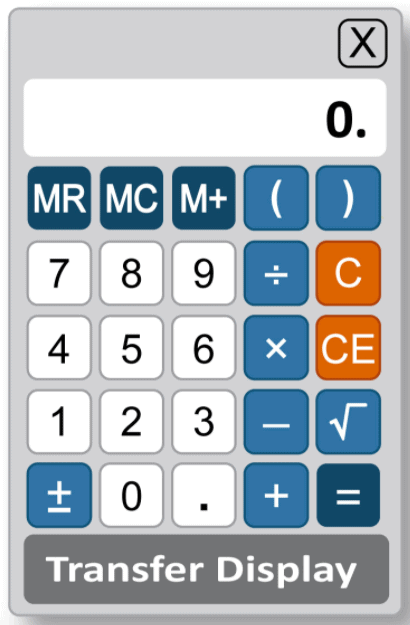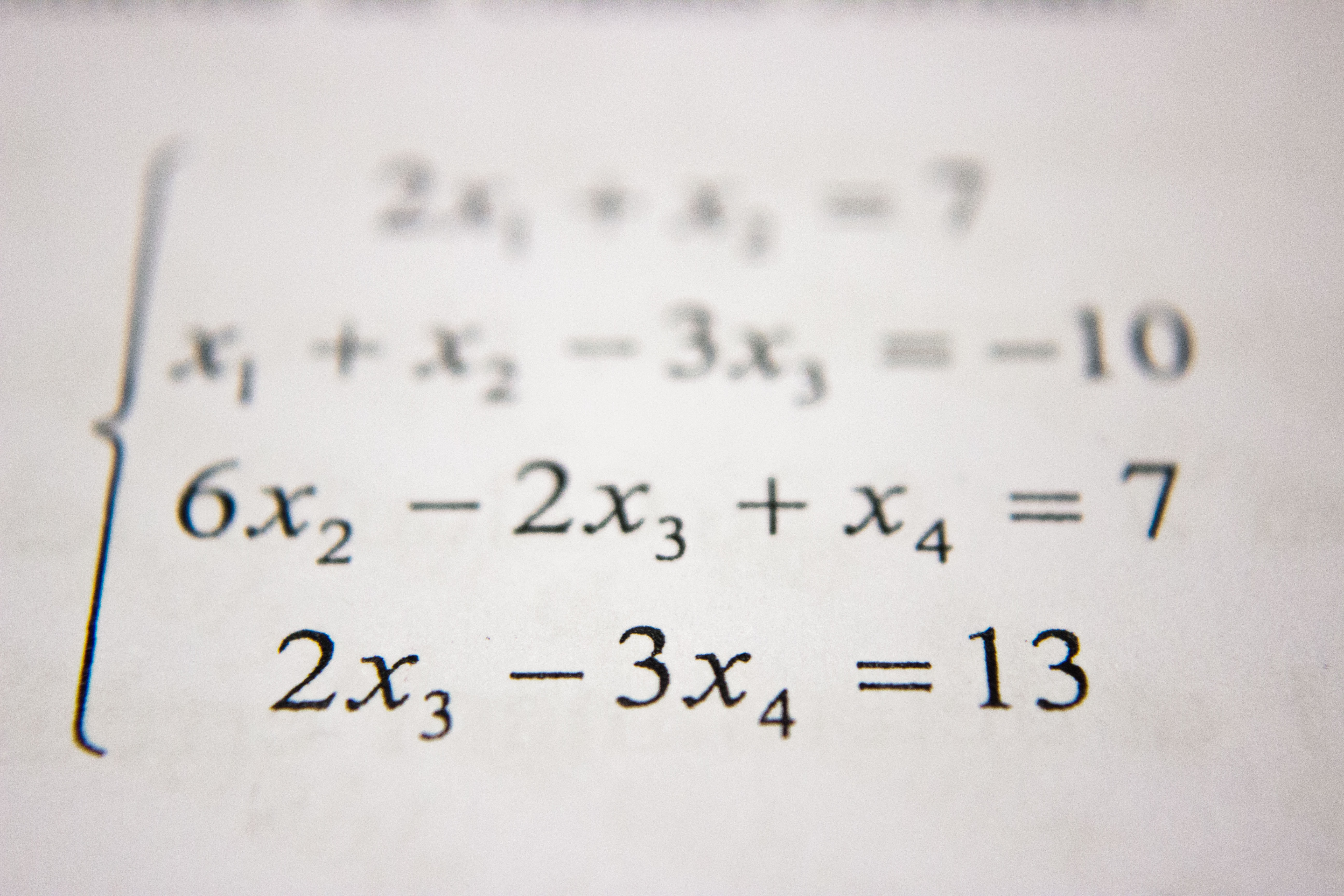GRE Exam

# All you need to know about the GRE calculatorScreenshot of the GRE calculator

You have access to a simple, on-screen calculator while taking the Quantitative Reasoning sections of the GRE (see example to the left). While the use of the calculator will help you by making computations faster, it is important to use it as quickly and efficiently as possible to avoid wasting your time.

Remember, the Quantitative Reasoning section measures is your overall mathematics knowledge. The answers provided to you by the GRE Calculator are to help you analyze the questions better.

You can try out the GRE Calculator online by getting PowerPrep by GRE test creator ETS.

## How does the GRE Calculator work?

The on-screen GRE calculator has four functions (addition, subtraction, multiplication and division) and a square root. The GRE calculator follows order of operations, which is a mathematical convention that establishes which operations are performed before others. Note that this is different from normal digital calculators which compute each function separately and immediately.

### Order of operations:

parentheses, exponentiation (including square roots), multiplications and divisions (from left to right), additions and subtractions (from left to right).

Example of order of operations:

The value of the expression 1 + 2 x 4 = 9

Why is the answer 9 and not 12?

Because the expression is evaluated as 2 x 4 = 8 first, and then + 1 to the result.

Remember, this is different from a normal digital calculator, which will do each calculation distinctly and immediately (it’d do 1+2 first, then do that result X 4 on your next calculation).

### Memory functions

The GRE Calculator also features one memory location and three memory buttons that function similar to basic calculators, which are:

• MR = Memory Recall
• MC = Memory Clear
• M+ = Memory Plus

Memory functions can be a great shortcut to faster calculations on the GRE. The M+ button adds a value to memory, where it will be stored as a number. Then, MR recalls that value for you to use again in another calculation. MC clears the stored value.Photo credit: Antoine Dautry on Unsplash

## When do you use the GRE Calculator?

The overall ease and speed of doing long mathematical computations with the use of a calculator will often be the number one reason for having one during the GRE test.

However, one of the best features that the GRE Calculator provides is its Transfer Display button. It is located at the bottom part and from the term itself, it allows transfer of calculator display to the answer box for Numeric Entry questions. This can save you valuable time and prevent mistakes.

## How do you properly make use of the GRE Calculator?

Ironically, the best way to prepare for using the GRE calculator is to minimize how often you need it.  You should avoid using the calculator for addition and subtraction, and most basic multiplication. Practice your multiplication tables (remember those?) and doing mental math.

Be very mindful of the calculator’s order of operations and remember to always start with the parentheses you’ll need to form the end formula. By doing so immediately, you can input the necessary computations much faster and avoid getting the order of operations wrong.

Lastly, avoid anything that would render an error message. Having to encounter such cases numerous times during the test will eat up your time, and are not necessary – no questions will go beyond the limits of the calculator’s abilities.

## Are there restrictions to the GRE Calculator?

Yes, the GRE calculator has the following restrictions:

• It can only display 8 digits
• It cannot complete computations that would not provide real numbers.
• It does not behave like a scientific calculator and has no functions for exponents, cubing, constants (like π or e), logarithmic (ln, log), or trigonometric (sine, cosine, tangent) functions.

We hope this guide helps you best use the calculator on the GRE exam. If you’re still considering study options for the GRE, check out Achievable’s GRE course – it’s mobile-friendly, easy to use, and our learning algorithm optimizes the course over time to give you the best results. It’s free to try, check out a chapter and see the Achievable difference for yourself.

Subscribe to our blog to get the latest GRE Exam tips, admissions advice, career advice, and more.

Join 160 other subscribers

We also post more great content on our social channels: